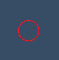# Is it possible to have different edgeColor and color on circleSource ?

Hi all,

I’m trying to colorize an actor whose mapper’s input connection is the output port of a circle source:

``````        var c = vtkCircleSource.newInstance();
c.setLines(true);
c.setFace(true);
c.setResolution(80)

const mapper = vtkMapper.newInstance();
mapper.setInputConnection(c.getOutputPort());
const actor = vtkActor.newInstance();
actor.getProperty().setColor(1, 1, 1);
``````

So far, so good.

But:

``````actor.getProperty().setEdgeColor(1, 0, 0);
``````

doesn’t work.

``````actor.getProperty().setLineWidth(3);
``````

doesn’t work either.

The question is then: is it possible to have at the same time different edge and face colors on actors build from circleSource?

In a different use case, the color is obtained from a lookup table:

``````        var lookupTable = vtkColorTransferFunction.newInstance();
const preset = vtkColorMaps.getPresetByName("rainbow");
lookupTable.applyColorMap(preset);
lookupTable.setMappingRange(0, 100);
lookupTable.updateRange();

actor.getMapper().setScalarModeToUseCellData();
actor.getMapper().setScalarRange(0, 100);
actor.getMapper().setLookupTable(lookupTable);

let d = vtkDataArray.newInstance({
name: "t",
dataType: 'Float32Array',
values: Array.from({ length: c.getOutputData().getPolys().getNumberOfCells() }, (v, i) => t)
});

let attr = vtkDataSetAttributes.newInstance();
attr.setScalars(d);
attr.setActiveScalars("t");
c.getOutputData().setCellData(attr);
``````

This was working well on spheres but is rendered differently with circle:So here only the edge is drawn and colored. Is that expected?